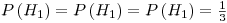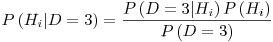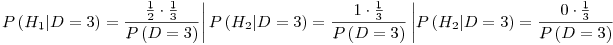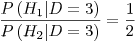# Hex, Bugs and More Physics | Emre S. Tasci

a blog about physics, computation, computational physics and materials…

## Bayesian Probabilities & Inference – Another example

### November 27, 2007 Posted by Emre S. Tasci

Yesterday, I told my battalion-buddy, Andy about the pregnancy example and, he recalled another example, with the shuffling of the three cups and a ball in one of them. The situation is proposed like this:

The dealer puts the ball in one of the cups and shuffles it so fast that at one point you lose the track and absolutely have no idea which cup has the ball. So, you pick a cup randomly (let’s say the first cup) but for the moment, don’t look inside. At this point, the dealer says, he will remove one of the remaining two cups and he guarantees you that, the one he removes does not have the ball. He even bestows you the opportunity to change your mind and pick the other one if you like. The question is this: Should you

a) Stick to the one you’ve selected or,

b) Switch to the other one or,

c) (a) or (b) – what does it matter?

Today, while I was studying MacKay’s book Theory, Inference, and Learning Algorithms, I came upon to the same example and couldn’t refrain myself from including it on this blog.

First, let’s solve the problem with simple notation. Let’s say that, initially we have picked the first cup, the probability that this cup has the ball is 1/3. There are two possibilities: Whether in reality the 1st cup has the ball indeed, or not.

i) 1st cup has the ball (1/3 of all the times): With this situation, it doesn’t matter which of the two cups remaining is removed.

ii) 1st cup doesn’t have the ball (2/3 of all the times): This time, the dealer removes the one, and the remaining cup has the ball.

So, to summarize, left with the two cups, there’s a 1/3 probability that the ball is within the cup we have chosen in the beginning while there’s the 2/3 probability that the ball is within the other cup. So, switching is the advantegous (did I spell it correctly? Don’t think so..) / To put it in other words: option (b) must be preffered to option (a) and actually, option (c) is wrong.

Now, let’s compute the same thing with the formal notations:

Let Hi be define the situation that the ball is in cup i. D denotes the removed cup (either 2 or 3). Now, initially the ball can be in any of the three cups with equal probabilities soNow, since we have selected the 1st cup, either 2nd or the 3rd cup will be removed. And we have three cases for the ball position:The first column represents that the ball is in our selected cup, so it doesn’t matter which of the remaining cups the dealer will remove. Each of the remaining cup has the same probability to be removed which is 0.5. The second column is the situation when the ball is in the second cup. Since it is in the second cup, the dealer will be forced to remove the third cup (ie P(D=3|H2)=1 ).Third column is similar to the second column.

Let’s suppose that, the dealer removed the third cup. With the 3rd cup removed, the probability that the ball is in the i. cup is given by: (remember that Hi represents the situation that the ball is in the i. cup)for our purposes, P(D=3) is not relevant, because we are looking for the comparison of the first two probabilities (P(D=3) = 0.5, by the way since it is either D=2 or D=3).meaning that it is 2 times more likely that the ball is in the other cup than the one we initially had selected.

Like John, Jo’s funny boyfriend in the previous bayesian example, used in context to show the unlikeliness of the "presumed" logical derivation, MacKay introduces the concept of one million cups instead of the three. Suppose you have selected one cup among the one million cups. Then, the dealer removes 999,998 cups and you’re left with the one you’ve initially chosen and the 432,238th cup. Would you now switch, or stick to the one you had chosen?